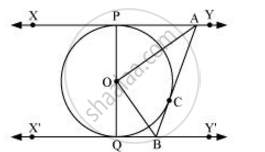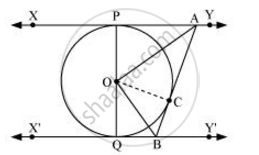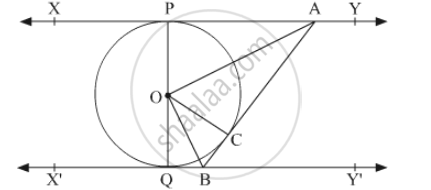# In the given figure, XY and X’Y’ are two parallel tangents to a circle with centre O and another tangent AB with point of contact C intersecting XY at A and X’Y’ at B. Prove that ∠AOB=90° - Mathematics

In the given figure, XY and X’Y’ are two parallel tangents to a circle with centre O and another tangent AB with point of contact C intersecting XY at A and X’Y’ at B. Prove that ∠AOB=90°#### Solution 1

Let us join point O to C.In ΔOPA and ΔOCA,

OP = OC (Radii of the same circle)

AP = AC (Tangents from point A)

AO = AO (Common side)

ΔOPA ≅ ΔOCA (SSS congruence criterion)

Therefore, P ↔ C, A ↔ A, O ↔ O

∠POA = ∠COA … (i)

Similarly, ΔOQB ≅ ΔOCB

∠QOB = ∠COB … (ii)

Since POQ is a diameter of the circle, it is a straight line.

Therefore, ∠POA + ∠COA + ∠COB + ∠QOB = 180º

From equations (i) and (ii), it can be observed that

2∠COA + 2 ∠COB = 180º

∠COA + ∠COB = 90º

∠AOB = 90°

#### Solution 2

Given: XY and X'Y' are two parallel tangents to the circle with centre O touching the circle at P and Q, respectively. AB is a tangent at the point C, which intersects XY at A and X'Y' at B.

To prove: ∠AOB = 90°

Construction: Join OC.In ΔOAP and ΔOAC,

OP = OC            (Radii of the same circle)

AP = AC             (Length of tangents drawn from an external point to a circle are equal)

AO = OA            (Common side)

ΔOAP ≅ ΔOAC    (SSS congruence criterion)

∴ AOP = COA       (C.P.C.T)        .....(1)

Similarly, ΔOBQ ≅ ΔOBC

∴ BOQ = COB                            .....(2)

POQ is a diameter of the circle. Hence, it is a straight line.

∴ AOP + COA + BOQ + COB = 180º

2COA + 2COB = 180º                              [From (1) and (2)]

⇒ COA + COB = 90º

⇒ AOB = 90°

Hence proved.

Concept: Number of Tangents from a Point on a Circle
Is there an error in this question or solution?

#### APPEARS IN

NCERT Class 10 Maths
Chapter 10 Circles
Exercise 10.2 | Q 9 | Page 214